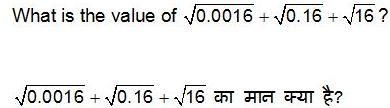# (NUMERICAL APTITUDE)

## ::NUMERICAL APTITUDE::

QID : 126 - What is the value of 12 – 22 + 32 – 42 + 62 – 52 + 82 – 72?
1) – 25
2) – 4
3) 4
4) – 16
QID : 127 - If A = 23 x 54 and B = 22 x 52 then what is the value of A x B?
1) 200000
2) 500000
3) 40000
4) 800000
QID : 128 - What is the smallest three digit perfect square?
1) 121
2) 100
3) 144
4) 128
QID : 129 -1) 4.44
2) 4.06
3) 4.04
4) 4.16
QID : 130 - What is the Least Common Multiple of 24, 60 and 48?
1) 120
2) 240
3) 180
4) 480
QID : 131 - What is the value of [(0.7)3 + (0.3)3] ÷ [(0.7)2– (0.7×0.3) + (0.3)2]?
1) 0.3
2) 0.7
3) 1
4) 0.4
QID : 132 - P and Q together complete a work in 8 days. Q and R together complete a work in 16 days and R and P together complete the same work in 8 days. In how many days P, Q and R together can complete the wok?
1) 6.4
2) 7.2
3) 5.8
4) 7.1
QID : 133 - U and V can complete a piece of work in 6 and 48 days respectively. They contracted to complete the work for Rs 45,000. What will be the share (in Rs) of U?
1) 35000
2) 37000
3) 36000
4) 40000
QID : 134 - The height of two cylinders are equal and their radius are in the ratio of 1 : 3 respectively. What is the respective ratio of the volume of the two cylinders?
1) 1 : 3
2) 1 : 9
3) 1 : 27
4) 1 : √3
QID : 135 - An article having marked price of Rs 4300 is sold at a discount of 17%. What is the selling price (in Rs) of the article?
1) 3721
2) 3459
3) 3569
4) 3317
QID : 136 - An article has marked price of Rs 6800. If two successive discounts of 12% and 20% are given, then what will be the selling price (in Rs)?
1) 4787.2
2) 4624
3) 4460.8
4) 4524

QID : 137 - Shopkeeper sold big, medium and small sized chairs for Rs 650, Rs 500 and Rs 400 respectively. The total number of chairs sold were in the ratio 8 : 13 : 4. What is the average cost (in Rs) of a chair?
1) 506
2) 546
3) 478
4) 532
QID : 138 - If u : v : w = 1 : 5 : 13, then what will be the value of (3u + 2v + 4w) / (2w – u – 4v)?
1) 25
2) 13
3) 12
4) 17
QID : 139 - What is the average of first 9 even numbers?
1) 9
2) 12
3) 11
4) 10
QID : 140 - There would be 20% loss if a toy is sold for Rs 11.20. At what price (in Rs) the toy should be sold to earn a profit of 10%?
1) 15.2
2) 14.8
3) 14.4
4) 15.4
QID : 141 - If the cost price of an article is 80% of the selling price, then what is the profit percent?
1) 20
2) 25
3) 30
4) 28
QID : 142 - What is the value of (42% of 7200) – (12% of 4800)?
1) 2138
2) 2448
3) 3348
4) 2918
QID : 143 - What is the value of 40% of 3/4 of 1200?
1) 360
2) 480
3) 120
4) 240
QID : 144 - A train crosses a platform in 50 seconds travelling with a speed of 72 km/hr. If the length of the train be 250 metres, then what will be the length (in metres) of the platform?
1) 600
2) 480
3) 750
4) 950
QID : 145 - Speed of a boat is 8 km/hr in still water and the speed of the stream is 2 km/hr. If the boat takes 8 hours to go to a place and come back, then what is the distance (in km) of the place?
1) 24
2) 30
3) 45
4) 42
QID : 146 - Rs 5000 are invested in a scheme of compound interest. If the rate of interest is 12% per annum, then what is the interest earned (in Rs) in 2 years?
1) 1450
2) 1272
3) 1520
4) 3450
QID : 147 -In the year 2014, the number of students of stream A is what percent of the number of students in stream B?
1) 75
2) 65
3) 62
4) 56
QID : 148 -In the year 2015, the number of students in stream B is how much percent more than the number of students in stream A?
1) 9
2) 10
3) 12
4) 18
QID : 149 -What is the average number of students in stream A from 2011 to 2015?
1) 612
2) 670
3) 586
4) 630